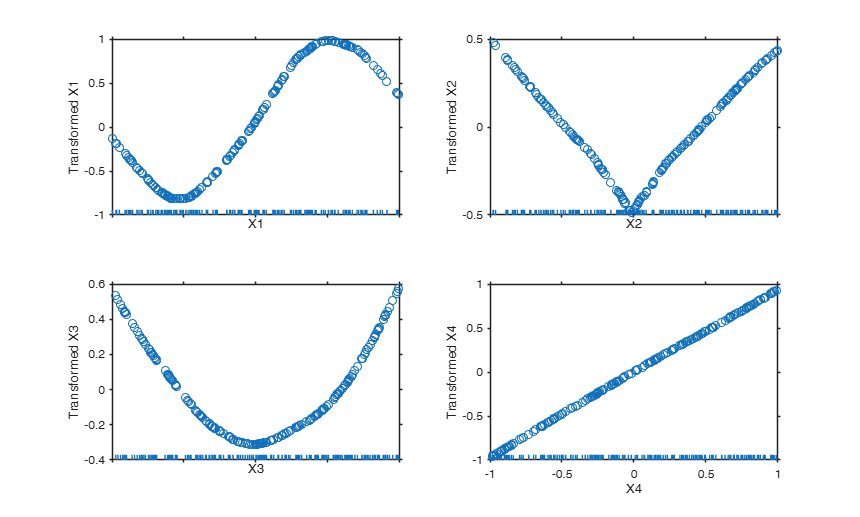# aceplot

aceplot produces the aceplot to visualize the results of ace

## Syntax

• aceplot(out)example
• aceplot(out,Name,Value)example

## Description

This function produces two figures. The first figure contains: the plot of transformed y vs. y (top left panel), the plot of residuals vs. fit (top right panel) and the plot of transformed y vs. fit (bottom left panel). The bottom right panel is left blank. This first figure is tagged pl_ty.

The second figure contains a series of p panels (where p is the number of columns of X) for transformed $X_j$ (tXj) vs. $X_j$ (with a rug plot along the tick marks). The second figure is tagged pl_tX.

These two figures can be combined in one figure, putting the plots of tXj vs $X_j$ in the bottom right panel of the first figure using optional input argument oneplot. If just one figure is produced it is tagged pl_tyX.

 aceplot(out) Example of the use of ace based on the Wang and Murphy data.

 aceplot(out, Name, Value) Example of use of option highlight.

## Examples

expand all

###Example of the use of ace based on the Wang and Murphy data.

In order to have the possibility of replicating the results in R using library acepack function mtR is used to generate the random data.

rng('default')
seed=11;
negstate=-30;
n=200;
X1 = mtR(n,0,seed)*2-1;
X2 = mtR(n,0,negstate)*2-1;
X3 = mtR(n,0,negstate)*2-1;
X4 = mtR(n,0,negstate)*2-1;
res=mtR(n,1,negstate);
% Generate y
y = log(4 + sin(3*X1) + abs(X2) + X3.^2 + X4 + .1*res );
X = [X1 X2 X3 X4];
% Apply the ace algorithm
out= ace(y,X);
% Show the output graphically using function aceplot
aceplot(out)### Example of use of option highlight.

load('illnessx07.txt');
y=illnessx07(:,4);
X=illnessx07(:,2:3);
p=size(X,2);
ycont=y;
listout=[17 53 30];
ycont(listout)=1;
l=[4*ones(p,1); 1];
outAC= ace(ycont,X,'l',l);
aceplot(outAC,'highlight',listout)

## Related Examples

expand all

### Example of the of option oneplot.

load('Marketing_Data')
y=Marketing_Data{:,4};
X=Marketing_Data{:,1:3};
% apply traditional avas (with all options set to false).
% Monotonicity of the expl. variables imposed.
out=avas(y,X,'l',3*ones(size(X,2),1))
% Put the plots of transformed Xj (tXj) agains Xj in the bottom right
% panel
aceplot(out,'oneplot',true)

## Input Arguments

### out — Structure containing the output coming from ace procedure. Structure.

Structure containing the following fields.

Value Description
ty

n x 1 vector containing the transformed y values.

tX

n x p matrix containing the transformed X matrix.

rsq

the multiple R-squared value for the transformed values in the last iteration of the outer loop.

y

n x 1 vector containing the original y values.

X

n x p matrix containing the original X matrix.

outliers

k x 1 vector containing the units declared as outliers when avas has been called with option rob set to trye.

Data Types: struct

### Name-Value Pair Arguments

Specify optional comma-separated pairs of Name,Value arguments. Name is the argument name and Value is the corresponding value. Name must appear inside single quotes (' '). You can specify several name and value pair arguments in any order as  Name1,Value1,...,NameN,ValueN.

Example:  'DataVars',[2 4] , 'highlight',1:10 , 'oneplot',true , 'notitle',true , 'VarNames',{'X1','X2', 'sales'} , 'ylimy', [-3 3; -2 2; -2 2] 

### DataVars —Variables for which $g(X_j)$ against $X_j$ has to be shown.vector of positive integers | [].

Columns of matrix X for which the plot of transformed value against original values needs to be computed. The default is empty, that is the plots are shown for all the columns of matrix X.

Example:  'DataVars',[2 4] 

Data Types: double

### highlight —units to highlight in the plot.vector.

Vector containing the numbers associated to the units to highlight in the plots.

The default is to highlight the units inside out.outliers.

Example:  'highlight',1:10 

Data Types: double

### oneplot —combined unique plot.boolean.

If oneplot is true just one figure is produced. The top left panel contains the plot of transformed y vs. y, the top right panel contains the plot of residuals vs. fit, the bottom left panel contains the plot of transformed y vs. fit. The bottom right panel contains a set of p subpanels (where p is the number of columns of X) for transformed $X_j$ (tXj) vs. $X_j$ (with a rug plot along the tick marks). If oneplot is false (default), the p panels for transformed $X_j$ (tXj) vs. $X_j$ are put in a separate figure.

Example:  'oneplot',true 

Data Types: logical

### notitle —no title in the plots.boolean.

If notitle is true the title in each panel is removed.

Note that the xlabel and ylabel in the various panels will still be present. The default is nottitle equal to false.

Example:  'notitle',true 

Data Types: logical

### VarNames —Names of the variabiles.empty value | string array | cell array of character vectors.

Names of variables specified as a string array or cell array of character vectors of length p+1, including the names for the columns of X first, and the name for the response variable y last.

If VarNames is empty {'X1','X2',...,'Xp','y'} (default).

Example:  'VarNames',{'X1','X2', 'sales'} 

Data Types: string array or cell array of character vector

### ylimy —2D array of size 3-by-2 which specifies the lower and upper limits for the 3 plots of the second figure.the first row refers to the plot of transformed y versus y, the second row refers to the plot of residuals versus fit and the third row to the the plot of transformed y versus fit.

The default value of ylimy is [], that is automatic scale is used.

Example:  'ylimy', [-3 3; -2 2; -2 2] 

Data Types: single | double

## Output Arguments

Breiman, L. and Friedman, J.H. (1985), Estimating optimal transformations for multiple regression and correlation, "Journal of the American Statistical Association", Vol. 80, pp. 580-597.

Wang D. and Murphy M. (2005), Identifying nonlinear relationships regression using the ACE algorithm, "Journal of Applied Statistics", Vol. 32, pp. 243-258.